Learning Library

# Two Digit Multiplication for 4th Grade

Ready to divide? Brush up on double digit multiplication skills beforehand with these fabulous worksheets.### 2-Digit Multiplication

Challenge your fourth grader with 2-digit multiplication. Give him a hand practicing multiplying double digits by double digits with this ninja worksheet.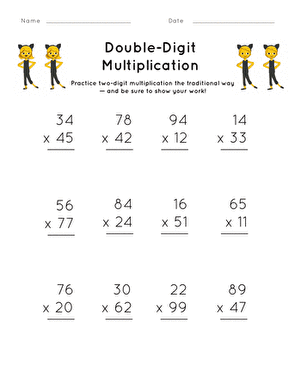### Double-Digit Multiplication

Your fourth grader can practice two-digit multiplication the traditional way with this practice sheet. Make sure they show their work!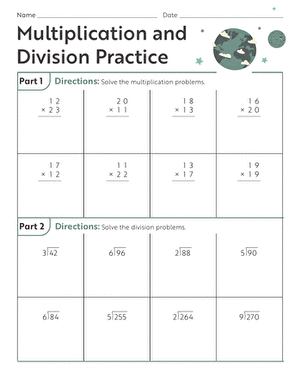### Multiplication and Division Practice Sheet #2

Put your child's math skills to the test with some two-digit multiplication and division practice problems.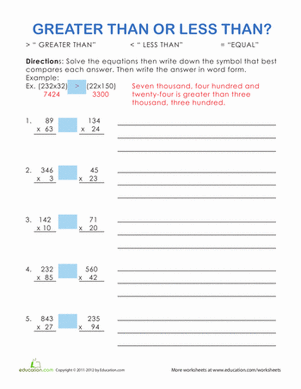### Greater Than or Less Than? #1

Do you remember your greater than or less than signs? Give your student some great practice solving long multiplication with this math worksheet.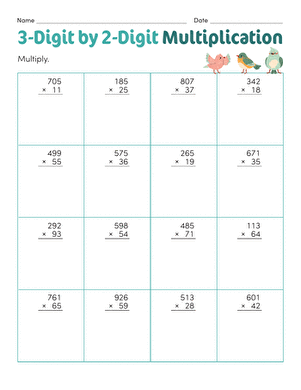### 3-Digit by 2-Digit Multiplication

Challenge your fourth grader with 3-digit by 2-digit multiplication. Practice multiplying triple digits by double digits with this exciting ninja worksheet.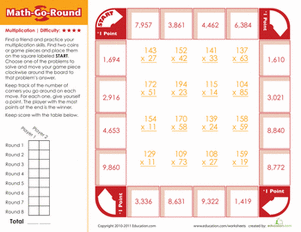### Math-Go-Round: Expert

Is your child an expert at multiplication? Find out with this fun, printable board game.

Create new collection

0

### New Collection>

0 items

What could we do to improve Education.com?